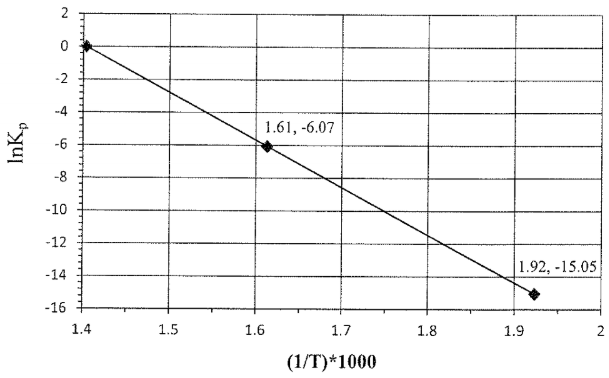# Problem: The temperature dependence of the equilibrium constant (Kp) is illustrated for the following reaction:NH4ClO4(s) → NH3(g) + HClO4(g)Note: the points are labeled as (1/T • 103, lnKp).Answer the following questions relating to the plot shown above:Calculate the partial pressure of NH3 (g) at 621 K if an excess amount of NH4ClO4 (s) is allowed to equilibrate in an evacuated closed vesel. Show all work and circle your final answer.

###### FREE Expert Solution

For this problem, we have to calculate the partial pressure of NH3 at 621 K if an excess amount of NH4ClOis allowed to equilibrate

To do so, we have to follow the steps:

Step 1. Analyze the graph and determine the value of Kp

Step 2. Calculate the equilibrium pressure of NH3

97% (495 ratings)###### Problem Details

The temperature dependence of the equilibrium constant (Kp) is illustrated for the following reaction:

NH4ClO4(s) → NH3(g) + HClO4(g)

Note: the points are labeled as (1/T • 103, lnKp).Answer the following questions relating to the plot shown above:

Calculate the partial pressure of NH3 (g) at 621 K if an excess amount of NH4ClO4 (s) is allowed to equilibrate in an evacuated closed vesel. Show all work and circle your final answer.